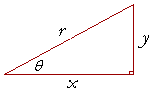Proof of the reciprocal identities

Proof of the tangent and cotangent identitiesThe proof of each of those follows from the definitions of the trigonometric functions, Topic 15.

Proof of the reciprocal relations

By definition:

 sin θ = yr , csc θ = ry .

Therefore, sin θ is the reciprocal of csc θ:

 sin θ = 1   csc θ

where 1-over any quantity is the symbol for its reciprocal; Lesson 5 of Algebra.  Similarly for the remaining functions.

Proof of the tangent and cotangent identities

To prove:

 tan θ  = sin θcos θ and cot θ  = cos θsin θ .

Proof.   By definition,

 tan θ  = yx .

Therefore, on dividing both numerator and denominator by r,

 tan θ = y/rx/r = sin θcos θ . cot θ = 1   tan θ = cos θsin θ .

Those are the two identities.

Proof of the Pythagorean identities

To prove:

 a) sin2θ + cos2θ = 1 b) 1 + tan2θ = sec2θ c) 1 + cot2θ = csc 2θ

Proof 1.   According to the Pythagorean theorem,x2 + y2 = r2.  .  .  .  .  . (1)

Therefore, on dividing both sides by r2,

 x2r2 + y2r2 = r2r2 =  1.

That is, according to the definitions,

cos2θ  +  sin2θ  =  1.  .  .  .  .(2)

Apart from the order of the terms, this is the first Pythagorean identity, a).

To derive b), divide line (1) by x2; to derive c), divide by y2.

Or, we can derive both b) and c) from a) by dividing it first by cos2θ and then by sin2θ.  On dividing line 2) by cos2θ, we haveThat is,

1 + tan2θ  =  sec2θ.

And if we divide a) by sin2θ, we haveThat is,

1 + cot2θ  =  csc2θ.

The three Pythagorean identities are thus equivalent to one another.

 Proof 2.sin2θ  + cos2θ = y2r2 + x2r2 = y2 + x2   r2 = r2r2 according to the Pythagorean theorem, = 1.

Trigonometric identities